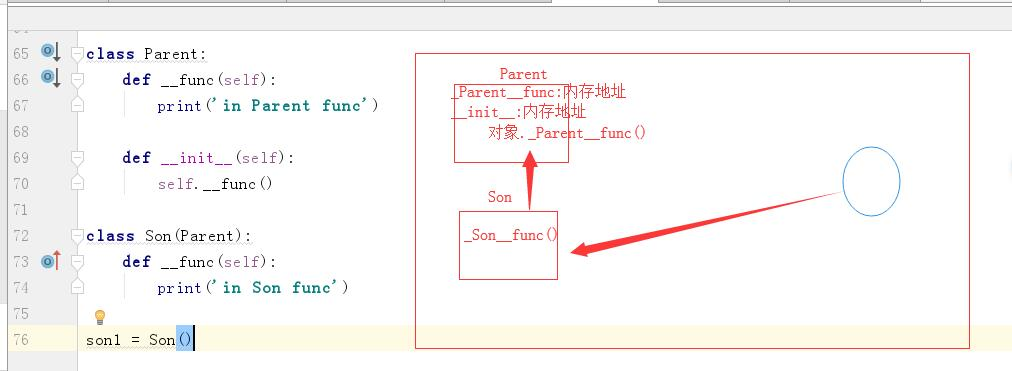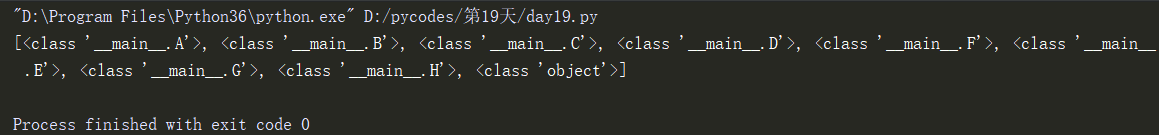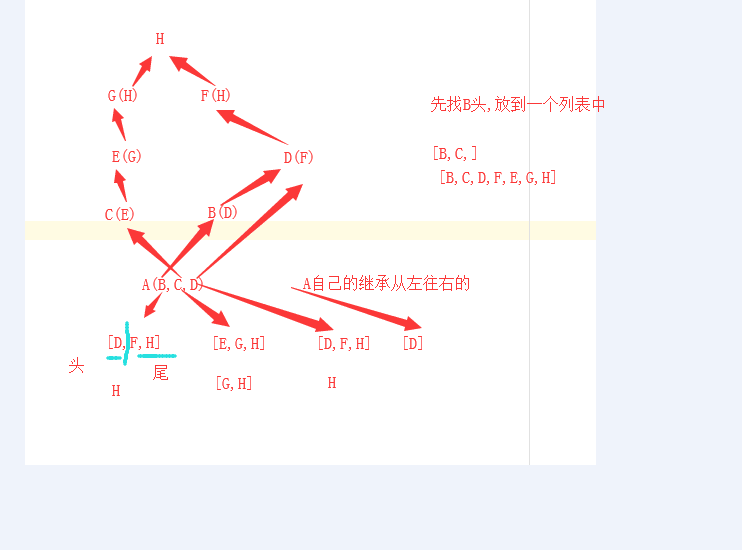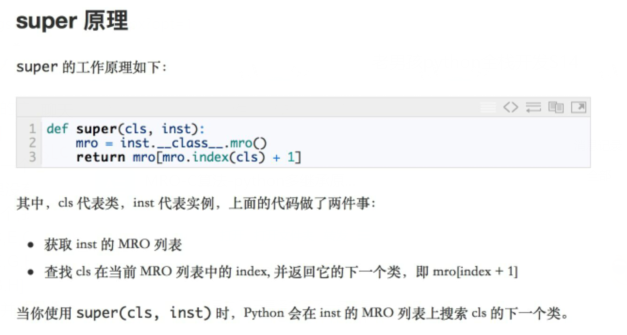## 面向对象--抽象类,多态,封装_weixin_30326741的博客-程序员秘密

```from abc import ABCMeta,abstractmethod
class Payment(metaclass=ABCMeta):  # 抽象类(接口类):
@abstractmethod
def pay(self): pass  # 制定了一个规范
class Alipay(Payment):
def __init__(self,money):
self.money = money

def pay(self):
print('使用支付宝支付了%s' %self.money)

class Jdpay(Payment):
def __init__(self, money):
self.money = money

def pay(self):
print('使用京东支付了%s' % self.money)

class Wechatpay(Payment):

def __init__(self,money):
self.money = money

def pay(self):
print('使用微信支付了%s' % self.money)

def pay(obj):
obj.pay()

w1 = Wechatpay(200)
a1 = Alipay(200)
j1 = Jdpay(100)
pay(a1)  # 归一化设计
pay(j1)

w1.Wechatpay()  #到用的时候会报错,因为已经强制执行def pay()函数的方法```
```接口提取了一群类共同的函数，可以把接口当做一个函数的集合。

`# python面向对象的三大特征之一:# 多态: python处处是多态.# java :强类型语言`
```# 这些类 都互称为鸭子.

class Str:
def index(self):
pass

class List:
def abc(self):
pass

class Tuple:
def rrr(self):
pass```

`# 广义的封装: 实例化一个对象,给对象空间封装一些属性.`
`# 狭义的封装: 私有制.`
`# 私有成员:私有静态字段,私有方法,私有对象属性`
```class B:
__money = 100000

class A(B):
name = 'alex'
__age = 1000

def func(self):
print(self.__age)
print(A.__age)    # 对于私有静态字段,类的内部可以访问.
print('func....')
def func1(self):
print(self.__money)
print(A.__money)
a1 = A()
print(a1.name)
print(A.name)

# print(a1.__age)  # 实例化对象不能访问私有静态字段
# print(A.__age)  # 类名不能访问私有静态字段```
`a1.func()  访问类的内部`
` `

```class B:
__money = 100000
def __f1(self):
print('B')

class A(B):
name = 'alex'

def __func(self):
print('func....')

def func1(self):
self.__func()   # 类的内部可以访问
self.__f1()   # 类的派生类也不能访问.
a1 = A()
a1.func1()  # 类的内部可以访问
a1.func1()  # 类的派生类也不能访问.```
`面试题`
```class Parent:
def __func(self):
print('in Parent func')

def __init__(self):
self.__func()

class Son(Parent):
def __func(self):
print('in Son func')

son1 = Son()`MRO-C算法-python多继承原理`
```class H:

def bar(self):
print('F.bar')
class G(H):

def bar(self):
print('F.bar')
class F(H):

def bar(self):
print('F.bar')
class E(G):

def bar(self):
print('E.bar')

class D(F):

def bar(self):
print('D.bar')

class C(E):
def bar(self):
print('C.bar')

class B(D):

def bar(self):
print('B.bar')

class A(B, C, D):

def bar(self):
print('A.bar')

a = A()
print(A.mro())```A(B,C,D)

L[B] = [B,D,F,H] #B往上面的继承顺序
L[C] = [C,E,G,H] #C往上面的继承顺序
L[D] = [D,F,H] #D往上面的继承顺序

L[A] = [B,C,D]

1 [B,D,F,H] [C,E,G,H] [D,F,H] [B,C,D]
2 [D,F,H] [C,E,G,H] [D,F,H] [C,D] #提取了头部的Ｂ，然后将其他列表头部的Ｂ删除，并将Ｂ放到list中
3 [D,F,H] [E,G,H] [D,F,H] [D] #因为第一个列表的D在其他列表的尾部存在,所以跳过D,然后找第二个列表的头部C,提取了头部的C，然后将其他列表头部的C删除，并将C放到list中

.......

4 [H] [H] [H] []

list = [A,B,C,D,F,E,G,H,object]### DB2-SQLSTATE,DB2-SQLCODE_42710 db2_自由自在_Yu的博客-程序员秘密

DB2-SQLSTATE,DB2-SQLCODE

### linux的文件指定查看工具-grep使用说明_magiclyj的博客-程序员秘密

1、使用说明：Linux系统中grep命令是一种强大的文本搜索工具，它能使用正则表达式搜索文本，并把匹 配的行打印出来。grep全称是Global Regular Expression Print，表示全局正则表达式版本，它的使用权限是所有用户。grep查找是按照行单位查找。2、使用格式：grep 关键字 文件(相对路径也可以) [选项/参数] grep [选项/参数] 关键字 文件(相对路径也可

### PHP CTF常见考题的绕过技巧_ctf php绕过_码啊码的博客-程序员秘密

1.===绕过PHP比较运算符 ===在进行比较的时候，会先判断两种字符串的类型是否相等，再比较值是否相等。只要两边字符串类型不同会返回false

### linux下一般程序运行时查找动态库的顺序_Bobsweetie的博客-程序员秘密

linux下一般程序运行时查找动态库的顺序：a.根据环境变量LD_LIBRARY_PATH查找b.根据/etc/ld.so.cache查找c.查找依次在/lib和/usr/lib目录查找。a 举例：由于动态库的链接和运行时的目录是分开指定的，所以编译通过后，运行时boost库找不到的问题存在于当前工程中。解决办法就是设置外环境变量LD_LIBRARY_PATH:#e

### 深入Android系统（十）PMS-2-初始化的一些细节_pms2-什么意思_apigfly的博客-程序员秘密

permission文件的处理在PMS的构造方法中初始化了PermissionManagerService，通过PermissionManagerService.create()方法，相关调用如下： public static PermissionManagerInternal create(......) { ...... new PermissionManagerService(context, defaultGrantCallback, externalLoc